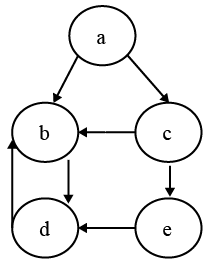# ISRO Scientist or Engineer Computer Science 2011

Instructions

For the following questions answer them individually

Question 61

# Three coins are tossed simultaneously. The probability that they will fall two heads and onefail isQuestion 62

# The average depth of a binary searchtree isQuestion 63

# What is the output of the following C code?#include <stdio.h>#include <conio.h>void main(){int index;for(index = 1; index <= 5; i++){printf("%d" , index);if(i == 3)continue;}}Question 64

# When n-type semiconductoris heated ?Question 65

# The Cyclomatic Complexity metric V(G) of the following control flow graph isQuestion 66

# Which of the following algorithm design techniques is used in merge sort?Question 67

# The arithmetic mean of attendance of 49 students of class A is 40% and that of 53 students of class B is 35%. Then the % of arithmeticn mean of attendance of class A and B isQuestion 68

# Which of the following sentences can be generated by$$S -> aS \mid bA$$$$A -> d \mid cA$$Question 69

# Light weight Directory Access Protocol is used forQuestion 70

# Numberof comparisons required for an unsuccessful search of an element in a sequential search organized, fixed length, symboltable of iength LisOR